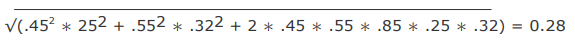# Quantitative Methods - Quantitative Methods Section 2

>>>>>>>>Quantitative Methods Section 2

 Security A B A 480 140 B 140 600

• A

0.26.• B

0.36.• C

0.46.• Option : A
• Explanation : Standard Deviation of A = √480= 21.91 Standard Deviation of B = √600 = 24.49 Corelation = 0.26

• A

16.7%.• B

22.2%.• C

27.0%.• Option : C
• Explanation : The total portfolio return is calculated as the weighted average return of the portfolio constituents. Portfolio return = (0.6 * 0.35) + (0.4 * 0.15) = 0.27 = 27.0%

 Fund A Fund B Portfolio weights (%) 45 55 Expected returns (%) 14 18 Standard deviations (%) 25 32 Correlation between thereturns of Fund A and Fund B 0.85

• A

17%.• B

28%.• C

35%.• Option : B
• Explanation : Calculate the portfolio standard deviation of returns as follows:Asset class Asset allocation (weight) (%) Asset class return (%) Correlation with equities class (%) Equities 65 22 100 Bonds 30 8 30 Cash and equivalents 5 1 25

• A

14.50%.• B

16.75%.• C

31.00%• Option : B
• Explanation : The portfolio return is the weighted mean return and is calculated as:0.65 * 22 + 0.30 * 8 + 0.05 * 1 = 16.75.

 Company Expected Return Standard Deviation Gala Cement 10% 8% Aqua Fertilizer 17% 20%

• Option : B
• Explanation : Systematic risk cannot be reduced.